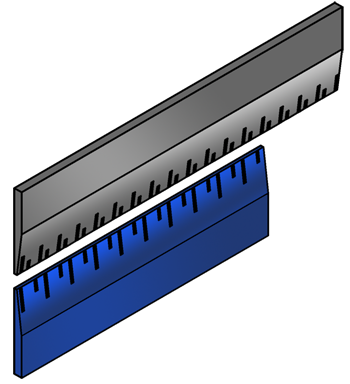# Length Unit Converter

Use this Conversion Calculator to convert between often used length units: inches, millimeters, nanometers, meters, foots, yards, miles.

## Initial data

Value: From:
To:Languages
Spanish (ES) Deutsch (DE)
Featured articles
How to read hydraulic circuits
Hydraulic cylinders
Hydraulic accumulators
Best calculators
Pressure loss calculator
Calculating the system curve
Hydraulic cylinder force calculator